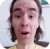FreeKB - Perl Hash (append values)
Perl - Hash (append values)Let's say you have the following hash. In this example, the employee_key in the hash is empty.

``my %hash = ( 'employee_key' => '' );``

You can count the elements.

``````my \$keys   = keys %hash;
my \$values = values %hash;
print "keys   = \$keys \n";
print "values = \$values \n";``````

Which will return 0, since employees_key is empty.

``````keys = 0
values = 0``````

Dumper can be used to display the structure of the hash.

``````use Data::Dumper;
print Dumper \%hash;``````

Which should produce the following.

``````\$VAR1 = {
'employees_key' => ''
};``````

A value can be appended to employee_key, like this.

``\$hash{"employee_key"} = "John Doe";``

Dumper can be used to display the structure of the hash.

``````use Data::Dumper;
print Dumper \%hash;``````

Now, employe_ key contains a value of John Doe.

``````\$VAR1 = {
'employee_key' => 'John Doe'
};``````

Multidimensional

In this example, name_key is below employee_key.

``my %hash = ( 'employee_key' => { 'name_key' => '' } );``

A value can be appended to name_key, like this.

``\$hash{employee_key}->{name_key} = "John Doe";``

Dumper can be used to display the structure of the hash.

``````use Data::Dumper;
print Dumper \%hash;``````

Now, name_key contains a value of John Doe.

``````\$VAR1 = {
'employee_key' => {
'name_key' => 'John Doe'
}
};``````

We will never share your name or email with anyone. Enter your email if you would like to be notified when we respond to your comment.

Please enter 7303e in the box below so that we can be sure you are a human.

Web design by yours truely - me, myself, and I   |   jeremy.canfield@freekb.net   |